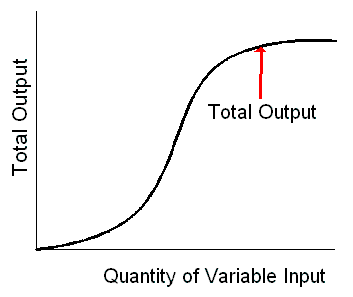### Why should I choose AnalystNotes?

AnalystNotes specializes in helping candidates pass. Period.

##### Subject 4. Marginal Returns and Productivity
A firm is an institution that hires factors of production and organizes them to produce and sell goods and services. Such factors (inputs) include land, labor, capital, and materials.

Productivity: The Relationship between Production and Cost

The total cost of production, TC = w x L + r x K, illustrates that the total cost is the cost of all the firm's inputs. The cost function, C = f(Q), is a relationship between the cost of production and the flow of output.

Two things determine the cost of production: the price and productivity. Production costs increase as input prices rise and fall as inputs become more productive. Input productivity is a measure of the output per unit of input.

Total, Average, and Marginal Product of Labor

Typical productivity measures for a firm are based on the concepts of total product, average product, and marginal product of labor.

• Total product of labor (TPL) is a short-run concept that is the total quantity that is able to be produced for each level of labor input, holding all other inputs constant.

The total product curve shows how total product changes with the quantity of variable input employed.Total product only provides insight into a firm's production volume relative to the industry; it does not show how efficient a firm is in producing its output.

• Average product of labor (APL) is the total product of labor divided by number of labor hours.

• Marginal product of labor (MPL), also known as marginal return, is the change in total product divided by the change in labor hours.

As more and more units of a variable resource are combined with a fixed amount of other resources, employment of additional units of the variable resource will eventually increase output only at a decreasing rate. Once diminishing returns are reached, it will take successively larger amounts of the variable factor to expand output by one unit.

The law of diminishing returns basically explains the old adage: "too many cooks spoil the broth," or too much of a good thing is bad. It dictates that additional output must fall as more and more labor is added to a fixed amount of capital.

Learning Outcome Statements

d. describe the phenomenon of diminishing marginal returns;

CFA® 2021 Level I Curriculum, , Volume 2, Reading 12

User Comment
space88 Don't understand the statement of "employment of additional units of the variable resource will eventually increase output only at a decreasing rate". Can someone pls help to explain?
micheleus it means that : output still increase but increase at decreasing rate.
Ex:
3 additional units of variable --> output increase 5
4 additional units of variable --> output increase 4
5 additional units of variable --> output increase 3
there you go.
jpducros There will be some question on the CFA exam about that LOS...know it well !
Thescholar Yes. This LOS shows that using more resources does not neccesarily mean higher output. This concept is as important as the priciples behind demand and supply
davcer In the real world not always happens that the more productive ones earn more
ajshittu I agree with davcer. However, the key words are "tend" and "skilled" and "unskilled".